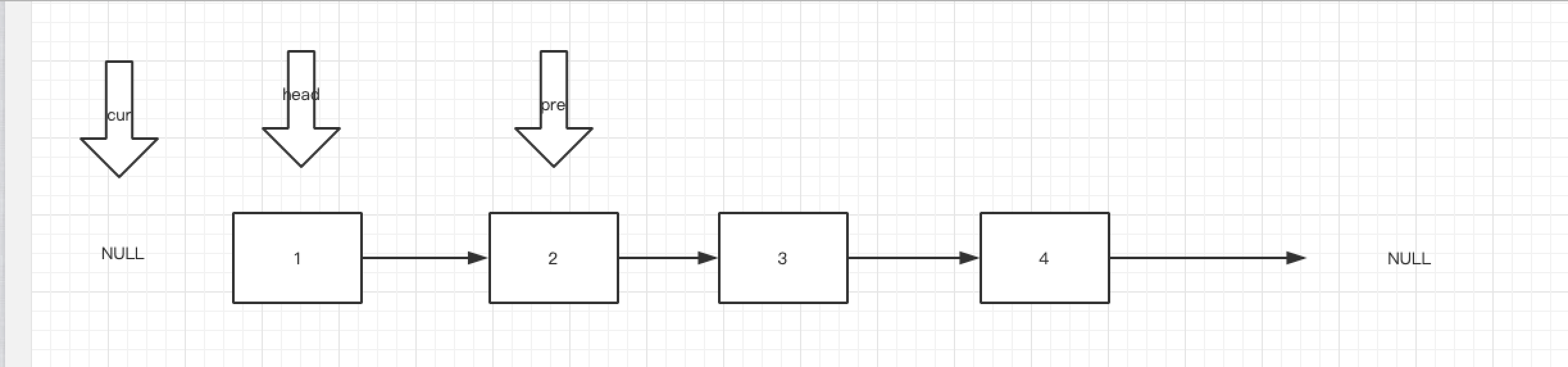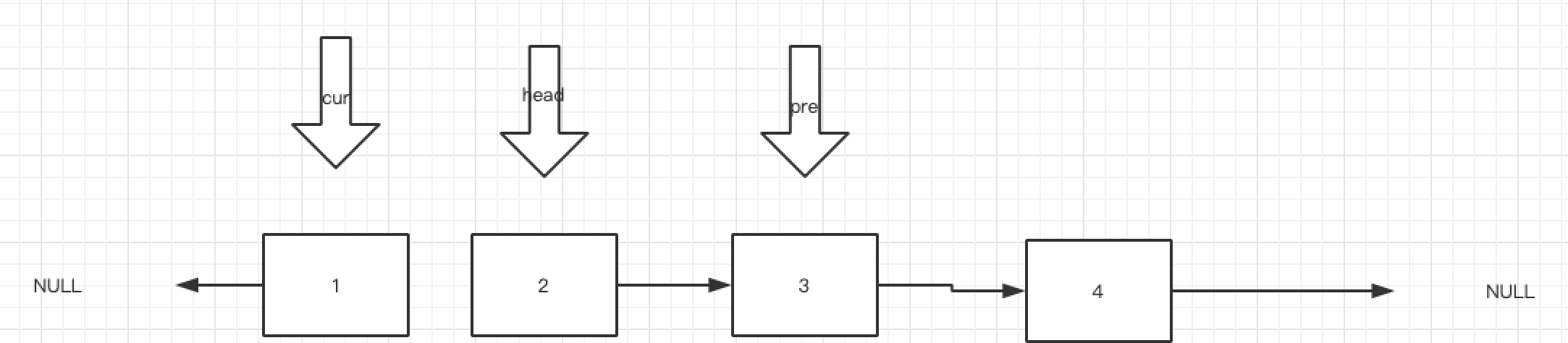# Go数据结构与力扣—链表

2021年4月9日 作者 y1nhui

# 前言

​ 因为想干纯后端，所以开始刷力扣巩固算法与数据结构，结果在写几天前的每日一题的时候发现自己对于链表的操作已经有些遗忘，以及对于链表的实现等也开始忘记。于是重新复习链表，并且写下这篇文章。

# 内容

## 基础知识

### 定义

``````type chainNode struct {
value int
next *chainNode
}
``````

### 头节点与头指针

``````type chainNode struct {
value int
next *chainNode
}

func main(){

chain := new(chainNode)

node1 := &chainNode{5,nil}
chain.next =node1

}

``````

### 单链表操作

#### 链表的创建

##### 尾插法

``````func createListTail(head *chainNode,n int) *chainNode{
for node := head ; node != nil ; node = node.next {
if node.next == nil {
node.next = &chainNode{n,nil}
break
}
}
}
``````

##### 头插法

``````func createListHead(head *chainNode,n int) *chainNode{
}
``````

#### 查找

``````func get(head *chainNode,n int) (int,error) {
i := 0
for node := head ; node != nil ; node = node.next{
if i==n {
return node.value,nil
}
i++
}

return 0,errors.New("Can't Find")
}
``````

#### 插入

``````func insert(head *chainNode,elem int,add int) (*chainNode,error) {
i := 0
for node:= head ; node != nil ; node = node.next {
newNode := &chainNode{elem,node.next}
node.next = newNode
}
i++
}

}
``````

#### 删除

``````func del(head *chainNode,n int)  {
i:=0
for node:=head;node != nil ; node = node.next {
if i == n-1 {
node.next = node.next.next
return
}
i++
}
}
``````

## 力扣题型

### 基础题

#### 231290.二进制链表转正数 难度：简单

``````func getDecimalValue(head *ListNode) int {
num := 0
for node := head ; node != nil ; node=node.Next {
num = num*2 +node.Val
}
return num
}
``````

#### 剑指offer 22 链表中倒数第K个节点 难度：简单

``````func getKthFromEnd(head *ListNode, k int) *ListNode {
n:=0
n++
}

for i:=1;i<=n-k+1;i++ {
cur = cur.Next
}
return cur
}
``````

``````func getKthFromEnd(head *ListNode, k int) *ListNode {
for ;k > 0; k -- {
fast = fast.Next
}
for fast != nil {
slow, fast = slow.Next, fast.Next
}
return slow
}
``````

#### 876.链表的中间节点 难度：简单

``````func middleNode(head *ListNode) *ListNode {
for fast.Next != nil && fast.Next.Next != nil {
fast=fast.Next.Next
low = low.Next
}
if fast.Next != nil {
return low.Next
}else {
return low
}
}
``````

#### 21.合并两个有序链表 难度：简单

``````func mergeTwoLists(l1 *ListNode, l2 *ListNode) *ListNode {
dummy := &ListNode{0,nil}
node := dummy
for l1 !=nil && l2!=nil {
if l1.Val < l2.Val {
node.Next = l1
node = node.Next
l1 = l1.Next
}else {
node.Next = l2
node = node.Next
l2 = l2.Next
}
}
switch {
case l1 != nil:
node.Next = l1
case l2 != nil:
node.Next = l2
}

return dummy.Next
}
``````

### 进阶题

#### 7.删除链表中的节点 难度：简单

``````func deleteNode(node *ListNode) {
*node = *node.Next
}
``````

#### 82.删除链表中的排序元素 难度：中等

``````func deleteDuplicates(head *ListNode) *ListNode {
for cur.Next != nil &&  cur.Next.Next != nil {
if cur.Next.Val == cur.Next.Next.Val{
v := cur.Next.Val
for cur.Next != nil && cur.Next.Val == v {
cur.Next = cur.Next.Next
}
}else {
cur = cur.Next
}
}

}
``````

#### 19.删除链表的倒数第N个节点 难度：中等

``````func removeNthFromEnd(head *ListNode, n int) *ListNode {
fast,slow := dummpy,dummpy
for n>=0 {
fast = fast.Next
n--
}
for fast != nil {
slow,fast = slow.Next,fast.Next
}
if slow.Next.Next!=nil{
slow.Next = slow.Next.Next
}else{
slow.Next = nil
}

return dummpy.Next
}
``````

#### 206.反转链表 && 剑指Offer 24 难度：简单``````func reverseList(head *ListNode) *ListNode {
var cur *ListNode
}
return cur
}
``````

#### 面试题02.07 链表相交 难度：简单

a \
o – c

b /

``````func getIntersectionNode(headA, headB *ListNode) *ListNode {
for p1 != p2 {
if p1 == nil {
} else {
p1 = p1.Next
}
if p2 == nil {
} else {
p2 = p2.Next
}
}
return p1
}

``````

#### 234.回文链表 难度：简单

​ 快慢指针找重点断开后做链表反转然后对比

``````func isPalindrome(head *ListNode) bool {
return true
}
var pre *ListNode

for fast!=nil && fast.Next != nil {
pre = slow
slow = slow.Next
fast = fast.Next.Next
}
pre.Next = nil

var dummy *ListNode
for slow != nil {
fast = slow.Next
slow.Next = dummy
dummy = slow
slow = fast
}

for dummy!=nil && head != nil {
return false
}
dummy = dummy.Next
}

return true
}
``````

#### 剑指offer 35.复杂链表的复制 难度：中等

``````func copyRandomList(head *Node) *Node {
return nil
}
for node!=nil {
newNode := &Node{
node.Val,
node.Next,
nil,
}
node.Next = newNode
node = newNode.Next
}

for node!=nil {
if node.Random != nil {
node.Next.Random = node.Random.Next
}
node = node.Next.Next
}

for node.Next != nil {
oldNode.Next = oldNode.Next.Next
node.Next = node.Next.Next
oldNode = oldNode.Next
node = node.Next
}
oldNode.Next = nil
}
``````

#### 142.环形链表2 难度：中等

``````func detectCycle(head *ListNode) *ListNode {
for fast != nil&&fast.Next != nil {   //考虑有指向nil这种阴间行为，记得.next!=nil
slow = slow.Next
fast = fast.Next.Next
if slow == fast{
for node != slow {
node = node.Next
slow = slow.Next
}
return node
}
}

return nil
``````

#### 148.排序链表 难度：中等

``````func sortList(head *ListNode) *ListNode {
l1 := []int{}
for node:=head;node != nil ;node = node.Next{
l1 = append(l1,node.Val)
}
sort.Ints(l1)
i:=0
node.Val = l1[i]
i++
}
}
``````

``````func sortList(head *ListNode) *ListNode {
if head == nil || head.Next == nil { // 递归的出口，不用排序 直接返回
}
var pre *ListNode
for fast != nil && fast.Next != nil {
pre = slow
slow = slow.Next
fast = fast.Next.Next
}
pre.Next = nil

l2 := sortList(slow)

return mergeList(l1,l2)
}

func mergeList(l1,l2 *ListNode) *ListNode {
dummy := &ListNode{0,nil}
node := dummy
for l1!=nil && l2!=nil {
if l1.Val < l2.Val {
node.Next = l1
l1 = l1.Next
}else {
node.Next = l2
l2 = l2.Next
}
node = node.Next
}
switch  {
case l1 != nil:
node.Next = l1
case l2 != nil:
node.Next = l2
}

return dummy.Next
}
``````